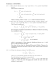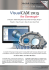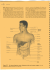Day 31

Transcription

Day 31
670 : by
Math
31
.
The
B the
foamy
Gun
reek TETPM ,
a
%In
diretm
,
,
geometry :
exppt ) to be the
define
edpntf
the
f lgkkl
geodesic
tfh
passing
&
T
gay
the
in
.
Jp . It )
if
other words
diﬀakl
in
B
in M
,z
geodes
a
st
&
8 pp 6) =p
.
0¥10 )
8
=
expplo )
than
,
=
Opel D
.
1*77
÷€
*
.
8pA A )
In
ymswtht
HW
ntie gps & ,
f
The Tf
6
.
Usfl
is
:
ft
(
lie
UAW
's
R
grip
's
UAP us
=
so
,
chrdeeopsithtnn
Pq
:
selx
& U
eh
"
Gin g
eigeveek
If
.
sue
A
=
f
=
,
Nw the diglwhes
,
if
,
wtke then eu
,
zMA÷l
:
mp exp g
.
in
me
than T
-
ft
& swjeete 12
defied
.
,
th
B
.
fate
I
6
→
with the Riemann
eqmehlnp at
coincides
the idly
also
"
=
,
'
so
the
'
'
n
A
A
'
.
ueau
=
.
as
.
'
a
.
+
U Tu
=
1h I
,
'
'
,
.
T
uhe
.
,
d
"
=
dist
U
et
u
akes
'
'
,
so
f et
EA &
one
is
mar
are
di
et he
At
-
trigtr
the eismrb
'
esnrotr for EA if egnwhe
an
It
uea a
=
eights f
the
,
Tiih
Th
jst
one
"
with
be
is
A
uE¥i
=
an
eA= eat
harm
f
in
ntrix A
X"
A 4 ezurhe Xi
UT Ut
well
B
the due eqmlt up
"
'
Ah
Ay gdx
nth A
is
then the matrix expulnl
:
eqmtl
504 )
→
:
trio
sqme
a
the mtrx
,
aht mtixeqatls
Lent : Tf A
If
mar
mtix
a
qymtlp fm SDH
nkix
the
ex
'
.
,
f
& U
ea
.
sex ?
egnudws
unity
Frth it
,
.
same
is
.
in
.
an
.
.
fr
As
€
Hit )
de
:
egrets
( R prklr
has
If
by
KAT
:
I
'
.
Hu
EA
,
the
In th
,
=
GUN , R )
→
it
ex T
=
a
.
In porter it
-
define
atzsmwtke
is
,
At
is
)
rotations
to
he
in
is
.
is
the
and
fixed axis
a
genet
if
B
:
fixed
a
axis
.
DID
=
axis
&
.
He
'
'
'
,
es
+
by
an
by the
A
is
&
A
She
define
lab d
(
.
5¥ A
It
=
5013 )
in
BE 5 DID
rows and
the
I¥ )
Es
=
Hood
t
e#B=eAeB
rotth and He
A comte
-
Mta
o=
"÷Q A
thin
2
0
afe
,
these
,
B
.
-
oto
and
time
eatf the
then the
eA*=e0
A
=
}!
Eg
( gal
-
if
are
,
sksyntid
&
eat
)
=
I
a
.
,
.
we
geodesy
canes
tht
see
poly
lines in
tfhtnihdif
gods ,z
in
SD
(3)
in Sds
-
she
this the
.
their
if
other
y
.
QEOH )
port
B
a
geometry
present way , geodesy
.
thh QEOH
are
fm Qeta
4¥
¥
he R
)
to
identity
)
San ) or an )
that
t.IQ
t.IQ
left troyKlm
,
now
EAT
so
A
'
th
,
A
let
5¥ A
Now read fm late I left tht if
So
=
A R B comte
It
.
exptt D= eta
of the
,
et
'
e
5013 )
→
ppb
B
se
is
.
Metal IR)
:
cos O
=
pp
Cose
.
*
"
"
'
tip
=
.
Sdl 3)
:
In
Of
.
if
shed tht
exp
Claim :
d
=
ex
'
Ah T
A
ya
Gail
:
e-
=
,
exp
,
'
=
title )
=
th
if AT XF
E Va IR
"
look
like
Q
4
ﬀ ]
[ OB
'
06
's
,
burn
went
her
we
geodesy in
& QE 04
Gets
'
whe AEOK ) & B
look like Q
or
then the
,
taot
peeps
to
"
Y ]
[ 93
"
tarot nets to
P
.
Ge R
"
the
Gam wrist
,
fr
B
restrict the
%¥
kxh
B
a
.
h
)
.
kxh -4 mtix
app sprite
choirs
.
of
d
:
"
Geodesistfh
) EKR
"
are
f
the
fm
HQ) et
I
& gwdesistyhtlalebdrn
z
are
fthefm
the )
Z
etl%% ]
.

Document 6427371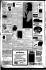Untitled - Exp.com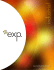Copy of 115L SYL spg 13.docx.docx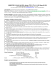E236N Tool PreSetter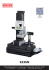inapasl n ialya hy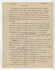MOTORCYCLE BAGS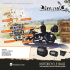Professional Indemnity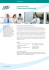TS60 industrial robot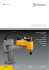Document 6514376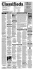arly students had it rough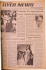Oil States Houma Machine Shop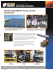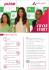PDF - Durham Research Online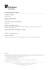JET FANS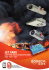1 - The Shandaken Project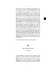An Example of Using a Rotary Table to Machine a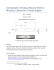Dexta Axis Melb launch:Dexta Axis Melb launch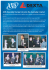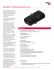I Hacked Your Network: Assessing I Hacked Your Network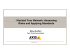Solutions 3 (STAT673)